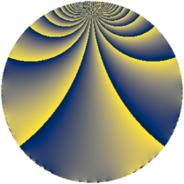# Properties

 Label 819.1.efLevel $819$ Weight $1$ Character orbit 819.ef Rep. character $\chi_{819}(451,\cdot)$ Character field $\Q(\zeta_{6})$ Dimension $2$ Newform subspaces $1$ Sturm bound $112$ Trace bound $0$

# Related objects

## Defining parameters

 Level: $$N$$ $$=$$ $$819 = 3^{2} \cdot 7 \cdot 13$$ Weight: $$k$$ $$=$$ $$1$$ Character orbit: $$[\chi]$$ $$=$$ 819.ef (of order $$6$$ and degree $$2$$) Character conductor: $$\operatorname{cond}(\chi)$$ $$=$$ $$91$$ Character field: $$\Q(\zeta_{6})$$ Newform subspaces: $$1$$ Sturm bound: $$112$$ Trace bound: $$0$$

## Dimensions

The following table gives the dimensions of various subspaces of $$M_{1}(819, [\chi])$$.

Total New Old
Modular forms 18 6 12
Cusp forms 2 2 0
Eisenstein series 16 4 12

The following table gives the dimensions of subspaces with specified projective image type.

$$D_n$$ $$A_4$$ $$S_4$$ $$A_5$$
Dimension 2 0 0 0

## Trace form

 $$2q - 2q^{4} + q^{7} + O(q^{10})$$ $$2q - 2q^{4} + q^{7} - q^{13} + 2q^{16} + 3q^{19} - q^{25} - q^{28} + 2q^{37} - q^{43} - q^{49} + q^{52} + 3q^{61} - 2q^{64} - 2q^{67} - 3q^{73} - 3q^{76} - 2q^{79} - 2q^{91} + 3q^{97} + O(q^{100})$$

## Decomposition of $$S_{1}^{\mathrm{new}}(819, [\chi])$$ into newform subspaces

Label Dim. $$A$$ Field Image CM RM Traces $q$-expansion
$$a_2$$ $$a_3$$ $$a_5$$ $$a_7$$
819.1.ef.a $$2$$ $$0.409$$ $$\Q(\sqrt{-3})$$ $$D_{6}$$ $$\Q(\sqrt{-3})$$ None $$0$$ $$0$$ $$0$$ $$1$$ $$q-q^{4}+\zeta_{6}q^{7}+\zeta_{6}^{2}q^{13}+q^{16}+(1+\cdots)q^{19}+\cdots$$